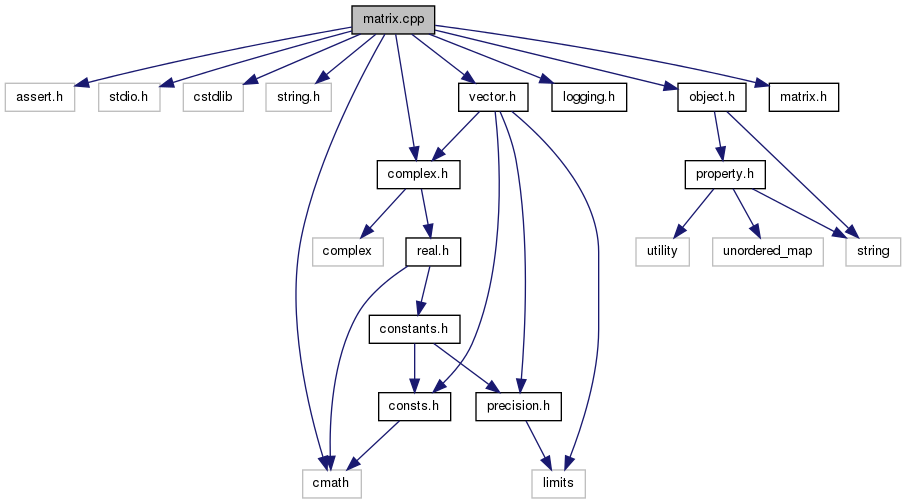Qucs-core  0.0.19
matrix.cpp File Reference

Dense matrix class implementation. More...

`#include <assert.h>`
`#include <stdio.h>`
`#include <cstdlib>`
`#include <string.h>`
`#include <cmath>`
`#include "logging.h"`
`#include "object.h"`
`#include "complex.h"`
`#include "vector.h"`
`#include "matrix.h"`
Include dependency graph for matrix.cpp:Go to the source code of this file.

namespace  qucs

## Functions

matrix qucs::operator+ (matrix a, matrix b)
Matrix addition.
matrix qucs::operator- (matrix a, matrix b)
Matrix subtraction.
matrix qucs::operator* (matrix a, nr_complex_t z)
Matrix scaling complex version.
matrix qucs::operator* (nr_complex_t z, matrix a)
Matrix scaling complex version (different order)
matrix qucs::operator* (matrix a, nr_double_t d)
Matrix scaling complex version.
matrix qucs::operator* (nr_double_t d, matrix a)
Matrix scaling real version (different order)
matrix qucs::operator/ (matrix a, nr_complex_t z)
Matrix scaling division by complex version.
matrix qucs::operator/ (matrix a, nr_double_t d)
Matrix scaling division by real version.
matrix qucs::operator* (matrix a, matrix b)
matrix qucs::operator+ (matrix a, nr_complex_t z)
Complex scalar addition.
matrix qucs::operator+ (nr_complex_t z, matrix a)
Complex scalar addition different order.
matrix qucs::operator+ (matrix a, nr_double_t d)
Real scalar addition.
matrix qucs::operator+ (nr_double_t d, matrix a)
Real scalar addition different order.
matrix qucs::operator- (matrix a, nr_complex_t z)
Complex scalar substraction.
matrix qucs::operator- (nr_complex_t z, matrix a)
Complex scalar substraction different order.
matrix qucs::operator- (matrix a, nr_double_t d)
Real scalar substraction.
matrix qucs::operator- (nr_double_t d, matrix a)
Real scalar substraction different order.
matrix qucs::transpose (matrix a)
Matrix transposition.
matrix qucs::conj (matrix a)
Conjugate complex matrix.
matrix qucs::adjoint (matrix a)
adjoint matrix
matrix qucs::abs (matrix a)
Computes magnitude of each matrix element.
matrix qucs::dB (matrix a)
Computes magnitude in dB of each matrix element.
matrix qucs::arg (matrix a)
Computes the argument of each matrix element.
matrix qucs::real (matrix a)
Real part matrix.
matrix qucs::imag (matrix a)
Imaginary part matrix.
matrix qucs::sqr (matrix a)
Multiply a matrix by itself.
matrix qucs::eye (int rs, int cs)
Create identity matrix with specified number of rows and columns.
matrix qucs::eye (int s)
Create a square identity matrix.
matrix qucs::diagonal (qucs::vector diag)
Create a diagonal matrix from a vector.
matrix qucs::pow (matrix a, int n)
nr_complex_t qucs::cofactor (matrix a, int u, int v)
Computes the complex cofactor of the given determinant.
nr_complex_t qucs::detLaplace (matrix a)
Compute determinant of the given matrix using Laplace expansion.
nr_complex_t qucs::detGauss (matrix a)
Compute determinant Gaussian algorithm.
nr_complex_t qucs::det (matrix a)
Compute determinant of the given matrix.
matrix qucs::inverseLaplace (matrix a)
Compute inverse matrix using Laplace expansion.
matrix qucs::inverseGaussJordan (matrix a)
Compute inverse matrix using Gauss-Jordan elimination.
matrix qucs::inverse (matrix a)
Compute inverse matrix.
matrix qucs::stos (matrix s, qucs::vector zref, qucs::vector z0)
S params to S params.
matrix qucs::stos (matrix s, nr_complex_t zref, nr_complex_t z0)
S renormalization with all part identic.
matrix qucs::stos (matrix s, nr_double_t zref, nr_double_t z0)
S renormalization with all part identic and real.
matrix qucs::stos (matrix s, qucs::vector zref, nr_complex_t z0)
S renormalization (variation)
matrix qucs::stos (matrix s, nr_complex_t zref, qucs::vector z0)
S renormalization (variation)
matrix qucs::stoz (matrix s, qucs::vector z0)
Scattering parameters to impedance matrix.
matrix qucs::stoz (matrix s, nr_complex_t z0)
Scattering parameters to impedance matrix identic case.
matrix qucs::ztos (matrix z, qucs::vector z0)
Convert impedance matrix scattering parameters.
matrix qucs::ztos (matrix z, nr_complex_t z0)
Convert impedance matrix to scattering parameters identic case.
matrix qucs::ztoy (matrix z)
impedance matrix to admittance matrix.
matrix qucs::stoy (matrix s, qucs::vector z0)
Scattering parameters to admittance matrix.
matrix qucs::stoy (matrix s, nr_complex_t z0)
Convert scattering pto adminttance parameters identic case.
matrix qucs::ytos (matrix y, qucs::vector z0)
Admittance matrix to scattering parameters.
matrix qucs::ytos (matrix y, nr_complex_t z0)
Convert Admittance matrix to scattering parameters identic case.
matrix qucs::stoa (matrix s, nr_complex_t z1, nr_complex_t z2)
Converts chain matrix to scattering parameters.
matrix qucs::atos (matrix a, nr_complex_t z1, nr_complex_t z2)
Converts chain matrix to scattering parameters.
matrix qucs::stoh (matrix s, nr_complex_t z1, nr_complex_t z2)
Converts scattering parameters to hybrid matrix.
matrix qucs::htos (matrix h, nr_complex_t z1, nr_complex_t z2)
Converts hybrid matrix to scattering parameters.
matrix qucs::stog (matrix s, nr_complex_t z1, nr_complex_t z2)
matrix qucs::gtos (matrix g, nr_complex_t z1, nr_complex_t z2)
matrix qucs::ytoz (matrix y)
Convert admittance matrix to impedance matrix.
matrix qucs::cytocs (matrix cy, matrix s)
Admittance noise correlation matrix to S-parameter noise correlation matrix.
matrix qucs::cstocy (matrix cs, matrix y)
Converts S-parameter noise correlation matrix to admittance noise correlation matrix.
matrix qucs::cztocs (matrix cz, matrix s)
Converts impedance noise correlation matrix to S-parameter noise correlation matrix.
matrix qucs::cstocz (matrix cs, matrix z)
Converts S-parameter noise correlation matrix to impedance noise correlation matrix.
matrix qucs::cztocy (matrix cz, matrix y)
Converts impedance noise correlation matrix to admittance noise correlation matrix.
matrix qucs::cytocz (matrix cy, matrix z)
Converts admittance noise correlation matrix to impedance noise correlation matrix.
matrix qucs::twoport (matrix m, char in, char out)
Generic conversion matrix.

## Detailed Description

Dense matrix class implementation.

References:

 Power Waves and the Scattering Matrix Kurokawa, K. Microwave Theory and Techniques, IEEE Transactions on, Vol.13, Iss.2, Mar 1965 Pages: 194- 202

 A Rigorous Technique for Measuring the Scattering Matrix of a Multiport Device with a 2-Port Network Analyzer John C. TIPPET, Ross A. SPECIALE Microwave Theory and Techniques, IEEE Transactions on, Vol.82, Iss.5, May 1982 Pages: 661- 666

 Comments on "A Rigorous Techique for Measuring the Scattering Matrix of a Multiport Device with a Two-Port Network Analyzer" Dropkin, H. Microwave Theory and Techniques, IEEE Transactions on, Vol. 83, Iss.1, Jan 1983 Pages: 79 - 81

 Arbitrary Impedance "Accurate Measurements In Almost Any Impedance Environment" in Scropion Application note Anritsu online(2007/07/30) http://www.eu.anritsu.com/files/11410-00284B.pdf

 Conversions between S, Z, Y, H, ABCD, and T parameters which are valid for complex source and load impedances Frickey, D.A. Microwave Theory and Techniques, IEEE Transactions on Vol. 42, Iss. 2, Feb 1994 pages: 205 - 211 doi: 10.1109/22.275248

 Comments on "Conversions between S, Z, Y, h, ABCD, and T parameters which are valid for complex source and load impedances" [and reply] Marks, R.B.; Williams, D.F.; Frickey, D.A. Microwave Theory and Techniques, IEEE Transactions on, Vol.43, Iss.4, Apr 1995 Pages: 914- 915 doi: 10.1109/22.375247

 Wave Techniques for Noise Modeling and Measurement S. W. Wedge and D. B. Rutledge, IEEE Transactions on Microwave Theory and Techniques, vol. 40, no. 11, Nov. 1992. pages 2004-2012, doi: 10.1109/22.168757 Author copy online (2007/07/31) http://authors.library.caltech.edu/6226/01/WEDieeetmtt92.pdf

Definition in file matrix.cpp.